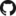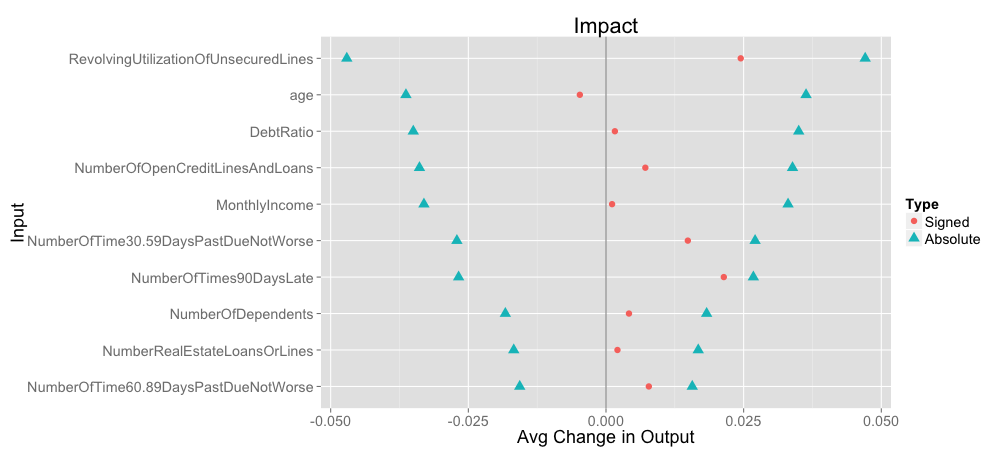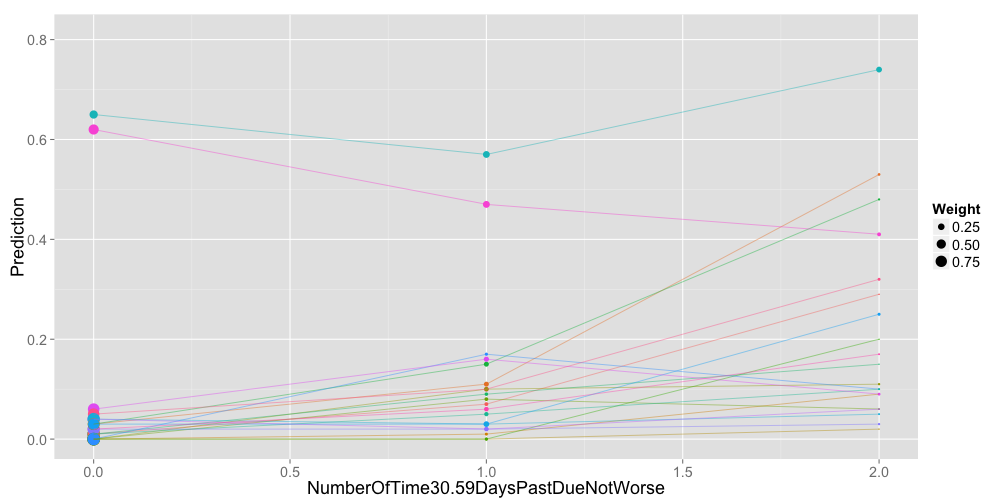# PredcompsHome

PDF Manual

Per Unit Input (original APC)

Impact (in units of output)

## Examples

Examples Overview

A fake logistic regression example predicting wine sales

A linear model with interactions

Loan defaults example

## More

As compared with Gelman & Pardoe 2007

Pairs & Weights

Please note: This is a very early version of the package. Please take it (and everything written on these pages) as provisional.

## An R Package for Understanding Arbitrary Complex Models

As complex models become widely used, it's more important than ever to have ways of understanding them. Even when a model is built primarily for prediction (rather than primarily as an aid to understanding), we still need to know what it's telling us. For each input to the model, we should be able to answer questions like these:

1. On average, much does the output increase per unit increase in input?
2. How does the influence of the input vary? (Across its range? In combination with other inputs?)
3. How much difference does variation in this input make to the predictions? (Relative to other inputs?)

For example, if our model were a linear regression with no interactions and no transformations of the inputs, the (1) would be answered by our regression coefficients, (2) would be answered “It doesn't vary”, and (3) would be a little harder but not too bad. All of these questions are much harder for more complicated models.

This R package is a collection of tools that are meant to help answer these questions for arbitrary complicated models. One advantage of the fact that they work work equally well for any model is that they can be used to compare models.

The key feature of the approach here is that we try to properly account for relationships between the various inputs.

### Origin

The ideas implemented here originate in Gelman and Pardoe 2007. If you are already familiar with the paper, you can skip to the differences between that and what's here. As far as I know, this is the only implementation intended for general use.

### Installation

The package is not hosted on CRAN, but it's still easy to install:

library(devtools)  # first install devtools if you haven't
install_github("predcomps", user = "dchudz")


### What we give you:

1. Average Predictive Comparisons Per Unit Input - On average, much does the output increase per unit increase in input? (This generalizes regression coefficients.)
2. Sensitivity Analysis - the package doesn't yet have functions that fully automate this, but see the bottom of this example for how this package can help with sensitivity analysis in a way that's consistent with accounting for relationships between the various inputs.
3. Impact - What is the average difference that a random change in each input makes to the predictions? (You can use this to compare the importance of the various inputs.)

You can compute both signed and absolute version of (1) and (3) for all inputs in your model with the function GetPredCompsDF and plot them using PlotPredCompsDF. See the PDF documentation for how to call each of these functions.

### Example Output

Here's an example of the output of impact (which gets at a idea similar to 'variable importance' in other packages) from an example predicting loan defaults. The model is a random forest. Note that impact is expressed in the units of the output variable (probabilities in this case), and hence it always makes sense to show it for all of the inputs on the same scale:The signed version should be interpreted as the expected value of the change in default probability for a random change in input. The absolute version is the expected absolute value, and is shown on both the positive and negative sides of horizontal axes. The signed version will always be between the two points representing the absolute version. When the signed version is close to the absolute version, the impact of a change the corresponding variable more consistently has the same sign.

We can also look in more detail at an individual input, examining the default probability as we vary that input (holding all else equal at a few example values):This is sometimes called a sensitivity analysis. Notice that in this case for one particular choice of other input values, transitioning from 0 to 1 previous time 30-59 days late leads to a decrease in predicted default probability. See the example for some thoughts on why this might be.

### Current Limitations

The package has some major limitations in its current form, but we have to start somewhere:

Input types: At the time of this writing, all inputs to the model must be numerical (or coercable to such). In practice this means that binary or ordered categorical inputs are okay, but unordered categorical inputs are not.

Parameters controlling the weights between pairs: These parameters are important for reasons that are both computational and statistical, are not yet well tuned for you, and the defaults may not be reasonable.

## Contact

I'm very interested in feedback from folks who are trying this out. Please get in touch with me at dchudz@gmail.com.